Find free online Chemistry Topics covering a broad range of concepts from research institutes around the world.

## Thermodynamics of Cell Reactions

We have just learnt that in a galvanic cell, the chemical energy is converted into electrical energy. The electrical energy produced by the cell is equal to the product of the total charge of electrons and the emf of the cell which drives these electrons between the electrodes.

If ‘n’ is the number of moles of electrons exchanged between the oxidising and reducing agent in the overall cell reaction, then the electrical energy produced by the cell is given as below.

Electrical energy = Charge of ‘n’ mole of electrons × Ecell ……. (9.20)
Charge of 1 mole of electrons = one Faraday (1F)
∴ Charge of ‘n’ mole of electrons = nF
Equation (9.20) ⇒ Electrical energy = nFEcell ……. (9.21)

∴ Charge of one elctron = 1.602 × 10-19C
∴ Charge of one mole of elctron = 6.023 × 1023 × 1.602 × 10-19C
= 96488 C
i.e., 1F = 96500 C

This energy is used to do the electric work. Therefore the maximum work that can be obtained from a galvanic cell is

(Wmax)cell = – nFEcell …………… (9.22)

Here the (-) sign is introduced to indicate that the work is done by the system on the surroundings.

We know from the Second Law of thermodynamics that the maximum work done by the system is equal to the change in the Gibbs free energy of the system.

i.e., Wmax = ∆G …………… (9.23)

From (9.22) and (9.23),

∆G = – nFEcell ……………. (9.24)

For a spontaneous cell reaction, the DG should be negative. The above expression (9.24) indicates that Ecell should be positive to get a negative ∆G value.

When all the cell components are in their standard state, the equation (9.24) becomes

∆G° = – nFE°cell ………….. (9.25)

We know that the standard free energy change is related to the equilibrium constant as per the following expression.

∆G° = – RTlnKeq …………… (9.26)
Comparing (9.25) and (9.26),
⇒ E°cell = $$\frac{2.303RT}{nF}$$log Keq ………….. (9.27)

Nernst Equation

Nernst equation is the one which relates the cell potential and the concentration of the species involved in an electrochemical reaction. Let us consider an electrochemical cell for which the overall redox reaction is,
xA + yB ⇄ lC + mD. The reaction quotient Q for the above reaction is given below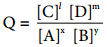……….. (9.28)

∆G = ∆G° + RT lnQ

The Gibbs free energy can be related to the cell emf as follows [∴equation (9.24) and (9.25)]
∆G = – nFE cell; ∆G° = – nFE°cell
Substitute these values and Q from (9.28) in the equation (9.29)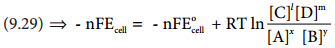………… (9.30)

Divide the whole equation (9.30) by (-nF)………… (9.31)

The above equation (9.31) is called the Nernst equation. At 25°C (298K), the above equation (9.31) becomes,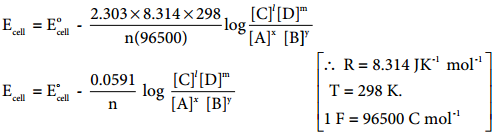……………… (9.32)

Let us calculate the emf of the following cell at 25°C using Nernst equation

Cu (s)|Cu2+(0.25 aq, M)|Fe2+(0.1 aq M)|Pt(s)
Given: (E°)Fe2+|Fe2+ = 0.77V and (E°)Cu2+|Cu = 0.34 V

Half reactions are
Cu (s) → Cu2+(aq) + 2e …………… (1)
2 Fe3+(aq) + 2e → 2Fe2+(aq) …………. (2)

the overall reaction is

Cu (s) + 2Fe3+(aq) → Cu2+(aq) + 2Fe2+ (aq), and n = 2
Apply Nernst equation at 25°C.Given standard reduction potential of Cu2+|Cu is 0.34V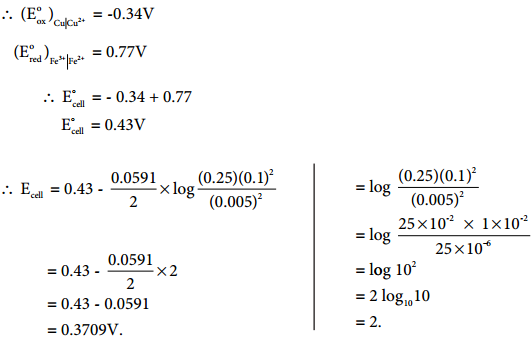Electrolytic Cell and Electrolysis

Electrolysis is a process in which the electrical energy is used to cause a non-spontaneous chemical reaction to occur; the energy is often used to decompose a compound into its elements. The device which is used to carry out the electrolysis is called the electrolytic cell.

The electrochemical process occurring in the electrolytic cell and galvanic cell are the reverse of each other. Let us understand the function of a electrolytic cell by considering the electrolysis of molten sodium chloride.

The electrolytic cell consists of two iron electrodes dipped in molten sodium chloride and they are connected to an external DC power supply via a key as shown in the figure (9.8). The electrode which is attached to the negative end of the power supply is called the cathode, and the one which attached to the positive end is called the anode. Once the key is closed, the external DC power supply drives the electrons to the cathode and at the same time pull the electrons from the anode.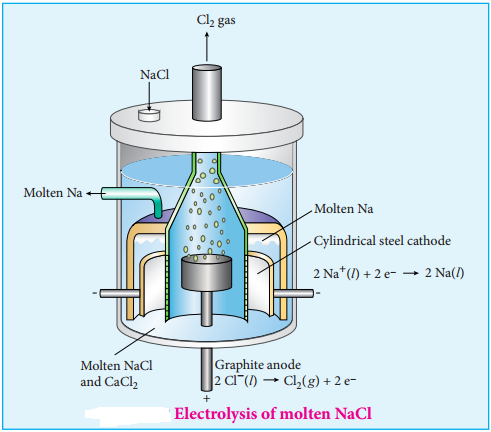Cell Reactions

Na+ ions are attracted towards cathode, where they combine with the electrons and reduced to liquid sodium.

Cathode (Reduction)

Na+(l) + e → Na(l) E° = – 2.71
Similarly, Cl ions are attracted towards anode where they lose their electrons and oxidised to chlorine gas.

Anode (Oxidation)

2Cl(l) → Cl2(g) + 2e E° = – 1.36V

The overall reaction is,
2Na+(l) + 2Cl(l) → 2Na(l) + Cl2(g) E° = – 4.07V

The negative E° value shows that the above reaction is a non spontaneous one. Hence, we have to supply a voltage greater than 4.07V to cause the electrolysis of molten NaCl. In electrolytic cell, oxidation occurs at the anode and reduction occur at the cathode as in a galvanic cell, but the sign of the electrodes is the reverse i.e., in the electrolytic cell cathode is – ve and anode is + ve.

First Law

The mass of the substance (m) liberated at an electrode during electrolysis is directly proportional to the quantity of charge (Q) passed through the cell.

i.e m α Q

We know that the charge is related to the current by the equation I = $$\frac{Q}{t}$$ ⇒ Q = It
∴ m α It
(or)
m = Z It …………. (9.33)

Where is Z is known as the electro chemical equivalent of the substance produced of the electrode. When, I = 1A and t = 1sec, Q = 1C, in such case the equation (9.32) becomes, (9.33)
⇒ m = Z …………….. (9.34)

Thus, the electrochemical equivalent is defined as the amount of substance deposited or liberated at the electrode by a charge of 1 coulomb.

Electro Chemical Equivalent and Molar Mass

Consider the following general electrochemical redox reaction
Mn+(aq) + ne → M(s)
We can infer from the above equation that ‘n’ moles of electrons are required to precipitate 1 mole of Mn+ as M(s).

The quantity of charge required to precipitate one mole of Mn+ = Charge of ‘n’ moles of electrons
= nF

In other words, the mass of substance deposited by one coulomb of charge…………… (9.35)

Second Law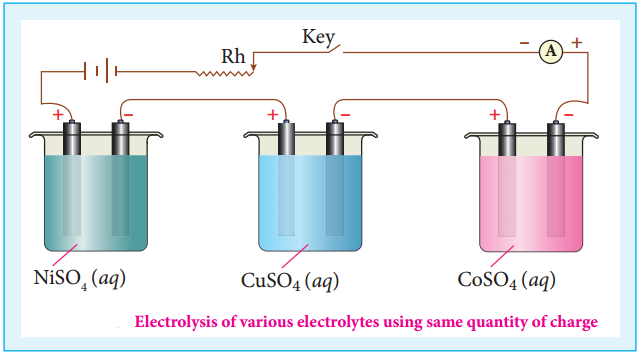When the same quantity of charge is passed through the solutions of different electrolytes, the amount of substances liberated at the respective electrodes are directly proportional to their electrochemical equivalents.

Let us consider three electrolytic cells connected in series to the same DC electrical source as shown in the figure 9.9. Each cell is filled with a different electrolytes namely NiSO4, CuSO4 and CoSO4, respectively.

When Q coulomb charge is passed through the electrolytic cells the masses of Nickel, copper and cobalt deposited at the respective electrodes be mNi, mCu and mCo, respectively.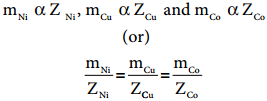………….. (9.36)

Batteries

Batteries are indispensable in the modern electronic world. For example, Li – ion batteries are used in cell phones, dry cell in flashlight etc. These batteries are used as a source of direct current at a constant voltage. We can classify them into primary batteries (non – rechargeable) and secondary batteries (rechargeable). In this section, we will briefly discuss the electrochemistry of some batteries.

Leclanche Cell

Anode: Zinc container
Cathode: Graphite rod in contact with MnO2
Electrolyte: ammonium chloride and zinc chloride in water

Emf of the cell is about 1.5V
Cell reaction

Oxidation at Anode
Zn (s) → Zn2+(aq) + 2e ……… (1)

Reduction at Cathode
2 NH4+(aq) + 2e → 2NH3(aq) + H2(g) ………… (2)

The hydrogen gas is oxidised to water by MnO2
H2 (g) + 2 MnO2 (s) → Mn2O3(s) + H2O(l)
Equation (1) + (2)+(3) gives the overall redox reactionZn (s) + 2NH4+(aq) + 2 MnO2(s) → Zn2+(aq) + Mn2O3 (s) + H2O(l) + 2NH3 ………… (4)

Ammonia produced at the cathode combines with Zn2+ to form a complex ion [Zn(NH3)4]2+(aq). As the reaction proceeds, the concentration of NH4+ will decrease and the aqueous NH3 will increase which lead to the decrease in the emf of cell.

Mercury Button Cell

Anode: zinc amalgamated with mercury
Cathode: HgO mixed with graphite
Electrolyte: Paste of KOH and ZnOOverall reaction: Zn (s) + HgO (s) → ZnO (s) + Hg (l)
Uses: It has higher capacity and longer life. Used in pacemakers, electronic watches, cameras etc.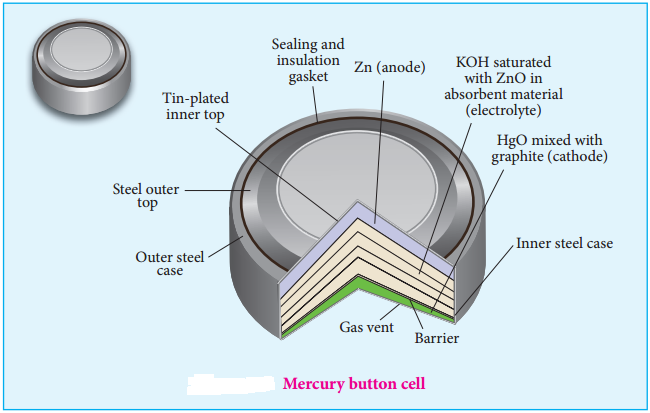Secondary Batteries

We have already learnt that the electrochemical reactions which take place in a galvanic cell may be reversed by applying a potential slightly greater than the emf generated by the cell. This principle is used in secondary batteries to regenerate the original reactants. Let us understand the function of secondary cell by considering the lead storage battery as an example.

Electrolyte: 38% by mass of H2SO4 with density 1.2g / mL.

Oxidation occurs at the anode

Pb(s) → Pb2+(aq) + 2e ……….. (1)
The Pb2+ ions combine with SO42-(aq) → PbSO4 (s) …………. (2)

Reduction occurs at the cathode

PbO2 (s) + 4 H+(aq) + 2e → Pb2+(aq) + 2H2O(l) ………. (3)
The Pb2+ ions also combine with SO42- → Pb2+(aq) + 2H2O(l)

The emf of a single cell is about 2V. Usually six such cells are combined in series to produce 12 volt

The emf of the cell depends on the concentration of H2SO4. As the cell reaction uses SO42-
ions, the concentration H2SO4 decreases. When the cell potential falls to about 1.8V, the cell has to be recharged.

Recharge of the Cell

As said earlier, a potential greater than 2V is applied across the electrodes, the cell reactions that take place during the discharge process are reversed. During recharge process, the role of anode and cathode is reversed and H2SO4 is regenerated.

Oxidation occurs at the cathode (now act as anode)

image

Reduction occurs at the anode (now act as cathode) PbSO4(s) + 2e → Pb(s) + SO42-(aq)
Overall reaction
2PbSO4(s) + 2H2O(l) → Pb(s) + PbO2(s) + 4H+(aq) + 2SO42-(aq)

Thus, the overall cell reaction is exactly the reverse of the redox reaction which takes place while discharging.

Uses:
Used in automobiles, trains, inverters etc.

The lithium – ion Battery

Anode: Porus graphite
Cathode: Transition metal oxide such as CoO2.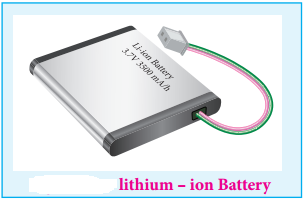Electrolyte: Lithium salt in an organic solvent
At the anode oxidation occurs
Li (s) → Li+(aq) + e

At the cathode reduction occurs
Li+ + CoO2(s) + e → LiCoO2(s)

Overall reactions
Li (s) + CoO2 → LiCoO2 (s)

Both electrodes allow Li+ ions to move in and out of their structures.

During discharge, the Li+ ions produced at the anode move towards cathode through the non – aqueous electrolyte. When a potential greater than the emf produced by the cell is applied across the electrode, the cell reaction is reversed and now the Li+ ions move from cathode to anode where they become embedded on the porous graphite electrode. This is known as intercalation.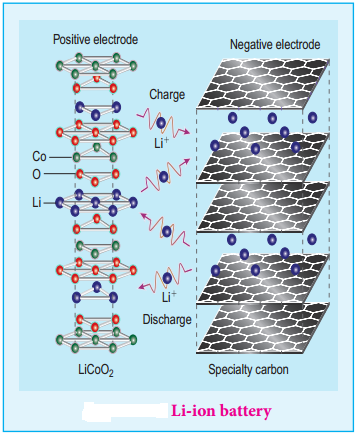Uses:

Used in cellular phones, laptops, computers, digital cameras, etc.

Fuel Cell:

The galvanic cell in which the energy of combustion of fuels is directly converted into electrical energy is called the fuel cell. It requires a continuous supply of reactant to keep functioning. The general representation of a fuel cell is follows.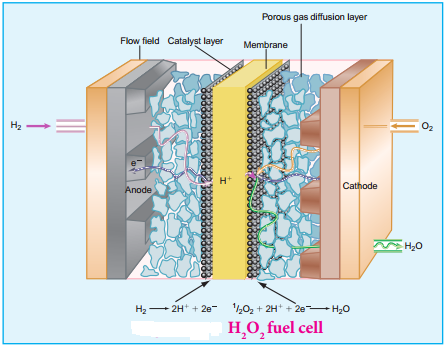Fuel | Electrode | Electrolyte | Electrode | Oxidant

Let us understand the function of fuel cell by considering hydrogen – oxygen fuel cell. In this case, hydrogen act as a fuel and oxygen as an oxidant and the electrolyte is aqueous KOH maintained at 200°C and 20 – 40 atm. Porous graphite electrode containing Ni and NiO serves as the inert electrodes.

Hydrogen and oxygen gases are bubbled through the anode and cathode, respectively.

Oxidation occurs at the anode:
2H2g + 4 OH(aq) → 4H2O(l) + 4e
Reduction occurs at the cathode O2(g) + 2H2O(l) + 4e → 4 OH(aq)

The overall reaction is 2H2 (g) + O2 (g) → 2H2O(l)

The above reaction is the same as the hydrogen combustion reaction, however, they do not react directly ie., the oxidation and reduction reactions take place separately at the anode and cathode respectively like H2 – O2 fuel cell. Other fuel cells like propane – O2 and methane O2 have also been developed.

Corrosion

We are familiar with the rusting of iron. Have you ever noticed a green film formed on copper and brass vessels?. In both, the metal is oxidised by oxygen in presence of moisture. This redox process which causes the deterioration of metal is called corrosion.

As the corrosion of iron causes damages to our buildings, bridges etc… it is important to know the chemistry of rusting and how to prevent it. Rusting of iron is an electrochemical process.

Electrochemical Mechanism of Corrosion

The formation of rust requires both oxygen and water. Since it is an electrochemical redox process, it requires an anode and cathode in different places on the surface of iron. The iron surface and a droplet of water on the surface as shown in figure (9.15) form a tiny galvanic cell. The region enclosed by water is exposed to low amount of oxygen and it acts as the anode.

The remaining area has high amount of oxygen and it acts as cathode. So based on the oxygen content, an electro chemical cell is formed corrosion occurs at the anode i,e,. in the region enclosed by the water as discussed below.At Anode (Oxidation):

Iron dissolves in the anode region

2Fe (s) → 2Fe2+ (aq) + 4e E° = 1.23V

The electrons move through the iron metal from the anode to the cathode area where the oxygen dissolved in water, is reduced to water.

At Cathode (Reduction):

The reaction of atmospheric carbon dioxide with water gives carbonic acid which furnishes the H+ ions for reduction.

O2(g) + 4H+(aq) + 4e → 2H2O(l) E° = 0.44 + 1.23 = 1.67V

The positive emf value indicates that the reaction is spontaneous.

Fe2+(aq) + O2(g) + 4H+(aq) → 4Fe3+(aq) + 2H2O(l)
4Fe3+(aq) + 4H2O(l) → Fe2O3.H2O(s) + 6H+(aq)

Other metals such as aluminium, copper and silver also undergo corrosion, but at a slower rate than iron. For example, let us consider the reduction of aluminium,
Al(s) → Al3+(aq) + 3e

Al3+, which reacts with oxygen in air to forms a protective coating of Al2O3. This coating act as a protective film for the inner surface. So, further corrosion is prevented.

Protection of Metals form Corrosion

This can be achieved by the following methods.

• Coating metal surface by paint.
• Galvanizing – by coating with another metal such as zinc. zinc is stronger reducing agent than iron and hence it can be more easily corroded than iron. i.e., instead of iron, the zinc is oxidised.
• Cathodic protection – In this technique, unlike galvanising the entire surface of the metal to be protected need not be covered with a protecting metal.

Instead, metals such as Mg or zinc which is corroded more easily than iron can be used as a sacrificial anode and the iron material acts as a cathode. So iron is protected, but Mg or Zn is corroded.

Passivation:

The metal is treated with strong oxidising agents such as concentrated HNO3. As a result, a protective oxide layer is formed on the surface of metal.

Alloy Formation:

The oxidising tendency of iron can be reduced by forming its alloy with other more anodic metals.
Example, stainless steel – an alloy of Fe and Cr.

Electrochemical Series

We have already learnt that the standard single electrode potentials are measured using standard hydrogen electrode. The standard electrode potential at 298K for various metal metal ion electrodes are arranged in the decreasing order of their standard reduction potential values as shown in the figure. This series is called electrochemical series.

The standard reduction potential (E°) is a measure of the oxidising tendency of the species. The greater the E° value, greater is the tendency shown by the species to accept electrons and undergo reduction. So higher the (E°) Value, lesser is the tendency to undergo corrosion.